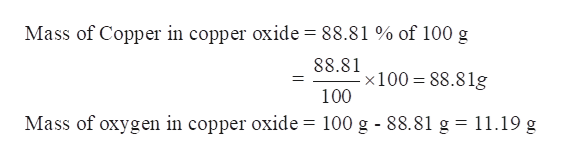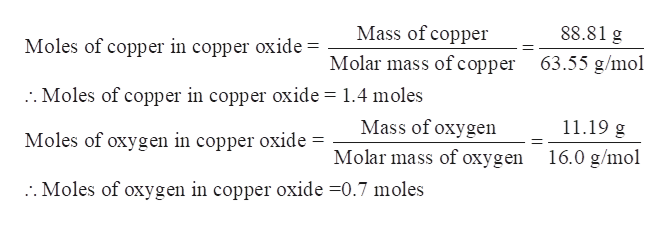A salt of copper and oxygen is 88.81% ( by mass) copper. Determine the empirical formula of the copper oxide salt.

Question

A salt of copper and oxygen is 88.81% ( by mass) copper. Determine the empirical formula of the copper oxide salt.

Step 1

A salt of copper and oxygen is 88.81% (by mass) copper. The empirical formula of the copper oxide salt is to be calculated.

Step 2

Determine the mass of copper and oxygen in the copper oxide-

Let the total mass of copper oxide taken = 100 g

Thus 88.81 % of this mass is copper and rest is oxygen.help_outlineImage TranscriptioncloseMass of Copper in copper oxide 88.81 % of 100 g 88.81 x100 88.81g 100 100 g 88.81 g = 11.19 g Mass of oxygen in copper oxide fullscreen
Step 3

Determine the moles of copper and oxygen in the copper oxide-

Molar mass of ...help_outlineImage TranscriptioncloseMass of copper 88.81 g Moles of copper in copper oxide = Molar mass ofcopper 63.55 g/mol 2. Moles of copper in copper oxide 1.4 moles Mass of oxygen 11.19 g Moles of oxygen in copper oxide Molar mass of oxygen 16.0 g/mol :. Moles of oxygen in copper oxide -0.7 moles fullscreen

Want to see the full answer?

See Solution

Want to see this answer and more?

Our solutions are written by experts, many with advanced degrees, and available 24/7

See Solution
Tagged in

General Chemistry